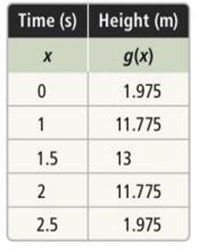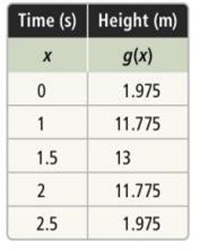Maths-
General
Easy

Question

# The balls are tossed up into the air . The function f(x)= -4.9x2 +14.7 x+0.975 models the path of Ball A. the path of ball B over time is shown in the table. Which ball reaches a greater height ? How much greater . Explain how you can answer without graphing either function .Hint:

## The correct answer is: 13 – 12 = 1

### Solution:- We have given two functions of ball A and ball B.f(x)= -4.9x2 +14.7 x+0.975We have to find the ball which reaches the greater height.For that we will find the vertex of both the functionsFor f(x) = -4.9x2 +14.7 x+0.975In f(x)= -4.9x2 +14.7 x+0.975,  a= -4.9, b= 14.7, and c= 0.975. So, the equation for the axis of symmetry is given byx = −(14.7)/2(-4.9)x = -14.7/-9.8x = 1.5The equation of the axis of symmetry for f(x)= -4.9x2 +14.7 x+0.975 is x = 1.5.The x coordinate of the vertex is the same:h = 1.5The y coordinate of the vertex is :k = f(h)k = -4.9h2 +14.7h +0.975k = -4.9(1.5)2 +14.7(1.5) + 0.975k = -11.025+22.05+0.975k = 12Therefore, the vertex of curve of ball A is (1.5 , 12)So , the maximum height of ball A is 12For ball B after observing the given table we have the maximum value of g(x) as 13, which is the maximum height of the ball.So, the ball B reaches at the greater height of 13.Difference between heights of ball A and ball B is 13 – 12 = 1#### With Turito Foundation.#### Get an Expert Advice From Turito.